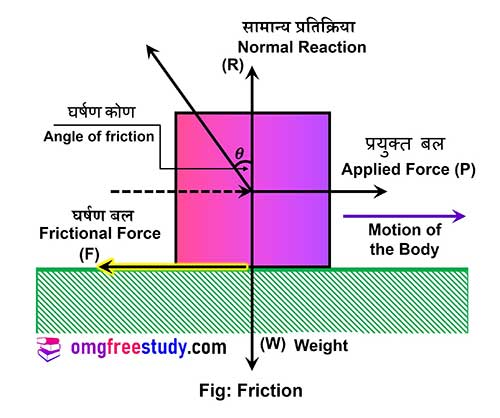# What is Friction? | Laws of Friction | Advantages

Obstruct the motion when one surface slide or tend to slide over the other surface is called as friction.

## Frictional Force Diagram

Consider a block is kept on a horizontal rough surface and just moved by the force (P) as shown in fig.Where,

P = Applied force

R = Normal reaction offered by the horizontal surface in upward direction.

W = Weight of block acts vertically downwards.

F = Frictional force.

• We can easily walk on the ground surface due to it.
• Vehicle moving on road can be stopped by applying brakes by producing sufficient frictional force.
• Hold writing material easily without slipping while writing on blackboard, slate or paper.
• One can hammer the nail into wall due to This.

• There is wear and tear to the surfaces of moving parts of various machine or equipment.
• Loss of energy due to friction therefore efficiency of machine reduce.
• Due to this consumption of fuel is more.
• It reduces speed of the moving object.

## laws of friction.

• The frictional force act tangential at the common surface in contact between the two bodies as shown in fig.
• And it always act in opposite direction to the direction of motion of a body.
• This frictional force can be increased up to limiting friction.
• When the body is just on the point motion.  It is in limiting equilibrium.
• If body is in limiting equilibrium, then ratio of limiting friction and normal reaction is constant, which is termed as coefficient of friction(μ).
• Static friction is always more than kinetic friction.

## Coefficient of Friction(μ)

Definition –

The ratio of limiting fric. to normal reaction at the surface of contact is a constant which is called as coefficient of fric. and it is denoted by letter ‘μ’.